• vs实现《数字图像处理与机器视觉》一书中图像旋转变换
作为代码界的菜鸟，最近在尝试着用vs实现《数字图像处理与机器视觉》一书中有关图像处理的VC++代码。目前先从简单的图像几何变换做起，希望能记录自己的成长^_^  本篇是图像旋转变换的实现。  代码中实现的是顺时针变换，如果想要逆时针变换，可以直接把角度设为负值，也可以在旋转函数中进行相应改变，改变方法可参照代码注释中变换方程式。  缺点是这个方法虽然显示了转换后的完整图像，但是旋转后显示的图像跟原图像相比，尺寸有所变化，目前还不知道怎么解决，希望在以后能够解决这个问题。  代码如下：
#include <iostream>
#include <mat.h>//头文件中增加了math.h
#include <opencv2\core\core.hpp>
#include <opencv2\highgui\highgui.hpp>
#include <opencv2\imgproc\imgproc.hpp>

using namespace std;
using namespace cv;
/********************************************
void imgRotate(Mat img, Mat &dst, float ang)
功能： 以原点为中心的图像旋转
注： 图像左上顶点顺时针旋转x1=x0*cost+y0*sint;y1=x0*(-sint)+y0*cost
图像左上顶点逆时针旋转x1=x0*cost-y0*sint;y1=x0*sint+y0*cost;
参数： Mat img：原图像
Mat dst：转置后图像
float ang: 旋转的角度
返回值： 无
*********************************************/
void imgRotate(Mat img, Mat &dst, float ang)
{
float pi = 3.1415926;
int nH = img.rows;
int nW = img.cols;
ang = ang*pi / 180;
int i, j, u, v,minx=0,miny=0,maxx=0,maxy=0;
//这个循环是为了确定旋转变换后原图像各像素对应的坐标，进而确定目标图像的大小，进而显示全部图像
for (i = 0; i < nH; i++)
{
for (j = 0; j < nW; j++)
{
u = int(i*cos(ang) + j*sin(ang) + 0.5);
v = int(-i*sin(ang) + j*cos(ang) + 0.5);
if (minx>u)
minx = u;
if (maxx < u)
maxx = u;
if (miny>v)
miny = v;
if (maxy < v)
maxy = v;
}
}
int dH = abs(minx)+maxx;
int dW = abs(miny)+maxy;
dst.create(dH, dW, img.type());
//注意这个循环的起始和终止条件
//判断目标图像的像素经过逆变换后的像素坐标在不在原图像中来确定目标图像像素值
for (i = minx; i < maxx; i++)
{
for (j = miny; j < maxy; j++)
{
u = int(i*cos(ang) - j*sin(ang) + 0.5);
v = int(i*sin(ang) + j*cos(ang) + 0.5);
if (u>0 && u<nH&&v>0 && v < nW)
dst.at<Vec3b>(i+abs(minx), j+abs(miny)) = img.at<Vec3b>(u, v);//i和j都加上最小行和列值的绝对值是因为目标图像的像素坐标均为正值
else
dst.at<Vec3b>(i + abs(minx), j + abs(miny)) = 0;
}
}
}
int main()
{
imshow("原图", img);
Mat dst;
//以图像左顶点为中心进行旋转变换
imgRotate(img, dst, 30);
cvNamedWindow("旋转变换",CV_WINDOW_FREERATIO);//这句是为了在显示结果图的时候可以自由控制结果图窗口的大小
imshow("旋转变换", dst);
waitKey(0);
return 0;
}
结果图如下：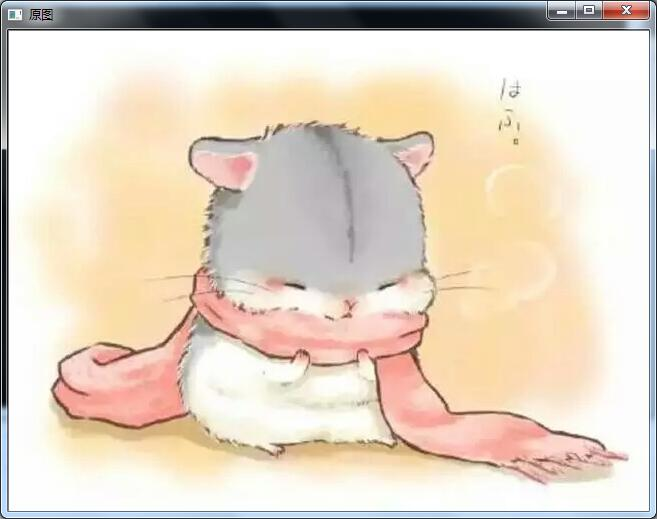顺时针30度旋转结果：逆时针30度旋转结果（角度设为-30）：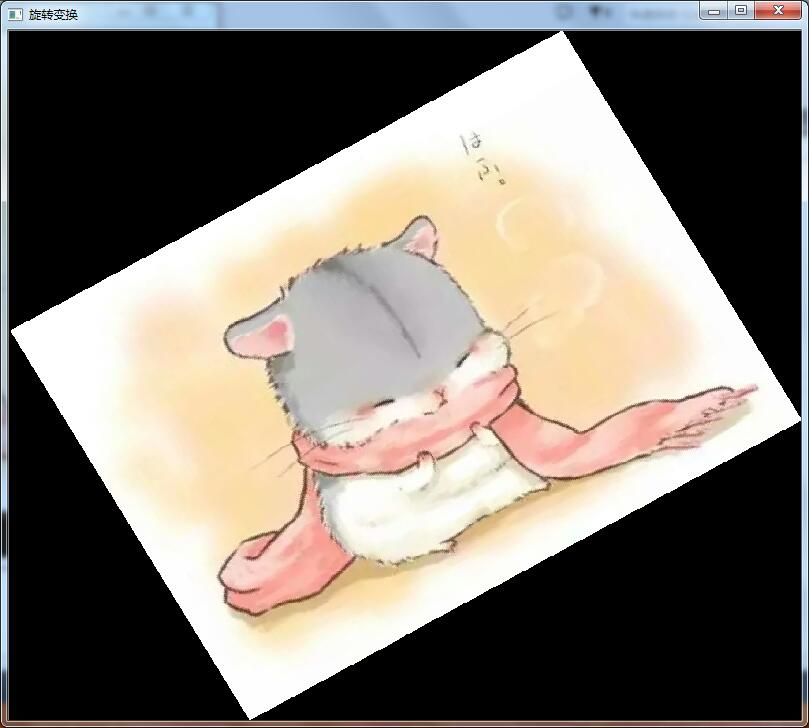在网上看到了一篇大牛实现图像旋转的文章，非常全面，涵盖了MATLAB实现，IplImage实现和Mat实现，文章链接如下：

图像旋转代码实现非常全的文章
展开全文vs
• 函数 cv2.getRotationMatrix2D((cols/2,rows/2),45,0.6) 第一个参数——原图像旋转...函数输出——仿射变换，2x3浮点矩阵 函数cv2.warpAffine(img,M,(2*cols,2*rows)) 第一个参数——原图像 第二个参数——2x3仿
函数 cv2.getRotationMatrix2D((cols/2,rows/2),45,0.6)  第一个参数——原图像旋转中心  第二个参数——以度为单位的旋转角度。正值表示逆时针旋转  第三个参数—— 各向同性的比例因子。  函数输出——仿射变换，2x3浮点矩阵  函数cv2.warpAffine(img,M,(2*cols,2*rows))  第一个参数——原图像  第二个参数——2x3仿射变换矩阵  第三个参数——生成图像的大小  函数cv2.getAffineTransform(pts1,pts2)  第一个参数——原图像中三角形的顶点坐标  第二个参数——变换后图像中三角形的顶点坐标  函数输出——两个坐标的变换矩阵
图像旋转
import cv2
import numpy as np
rows,cols = img.shape
M = cv2.getRotationMatrix2D((cols/2,rows/2),45,0.6)
dst = cv2.warpAffine(img,M,(2*cols,2*rows))
while(1):
cv2.imshow('img',dst)
if cv2.waitKey(1)&0xFF == 27:
break
cv2.destroyAllWindows()
图像放射变换
import cv2
import numpy as np
from matplotlib import pyplot as plt

rows,cols,ch = img.shape
pts1 = np.float32([[50,50],[200,50],[50,200]])
pts2 = np.float32([[10,100],[200,50],[100,250]])
M = cv2.getAffineTransform(pts1,pts2)
dst = cv2.warpAffine(img,M,(cols,rows))

plt.subplot(121)
plt.imshow(img)
plt.title('input')
plt.subplot(122)
plt.title('output')
plt.imshow(dst)
plt.show()
图像透视变换
import cv2
import numpy as np
from matplotlib import pyplot as plt

rows,cols,ch = img.shape

pts1 = np.float32([[56,65],[368,52],[28,387],[389,398]])
pts2 = np.float32([[0,0],[300,0],[0,300],[300,300]])

M = cv2.getPerspectiveTransform(pts1,pts2)
dst = cv2.warpPerspective(img,M,(300,300))

plt.subplot(121)
plt.imshow(img)
plt.title('input')
plt.subplot(122)
plt.title('output')
plt.imshow(dst)
plt.show()
展开全文python opencv
• 转：图像旋转变换的推导 前面我们提到了图像的缩放变换，可以用矩阵乘法的形式来表达变换后的像素位置映射关系。 那么，对于旋转变换呢？我们可以同样将其想象成二维平面上矢量的旋转。如下图所示，矢量[x1,y1]逆...
转：图像旋转变换的推导
前面我们提到了图像的缩放变换，可以用矩阵乘法的形式来表达变换后的像素位置映射关系。
那么，对于旋转变换呢？我们可以同样将其想象成二维平面上矢量的旋转。如下图所示，矢量[x1,y1]逆时针旋转θθ度到了[x2,y2]。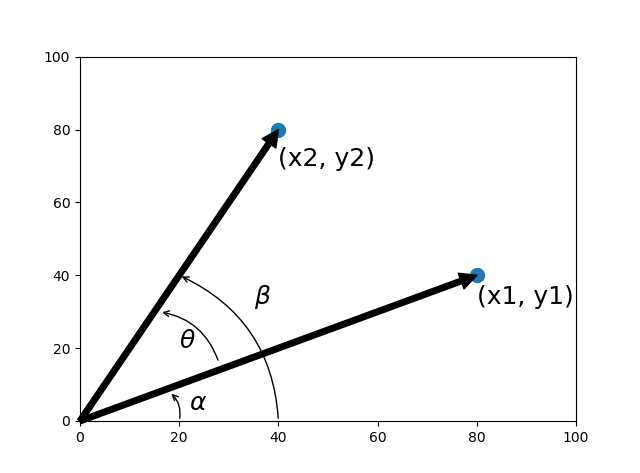设定矢量的长度为s，根据坐标系定义，我们可以得到：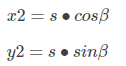根据上面的图形，有：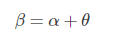因此: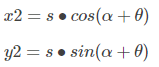根据初中所学的三角函数公式：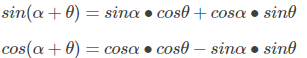于是：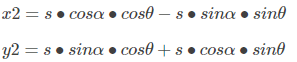由于：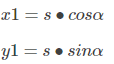因此：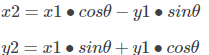于是，上式写成矩阵乘法的形式如下：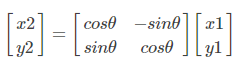我们来看看一个图像逆时针旋转180度的情况。
import cv2

import numpy as np
import math

row, col = lenna.shape

lenna_rotation = np.zeros_like(lenna)

A = np.mat([[math.cos(math.pi), -math.sin(math.pi)], [math.sin(math.pi), math.cos(math.pi)]])

for r in range(row):
for l in range(col):
v = np.dot(A.I, np.array([r, l]).T)

lenna_rotation[r, l] = lenna[int(v[0, 0]), int(v[0, 1])]

cv2.imshow("lenna", lenna)
cv2.imshow("rotation", lenna_rotation)
cv2.waitKey()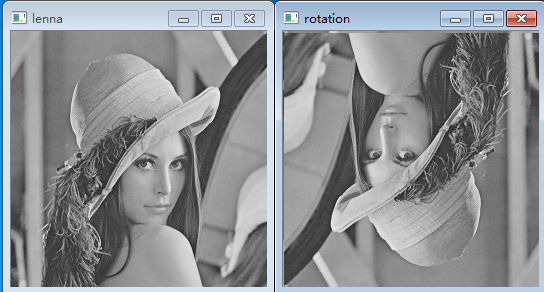上面的图像宽度和高度是一样的，而且旋转角度是180度，比较特殊。在一般情况下，我们需要注意的是2点：一是旋转图像一般要将旋转中心设置在图像的中心点位置；二是图像旋转后，可能越过了原来的图像边界范围。这些都比较麻烦，好在opencv已经做好了这一切。
lenna = cv2.imread("lenna256.png", 0)
row, col = lenna.shape
M = cv2.getRotationMatrix2D((col // 2, row // 2), 70, 0.5)
dst = cv2.warpAffine(lenna, M, (col, row))
cv2.imshow("rotation", dst)
cv2.waitKey()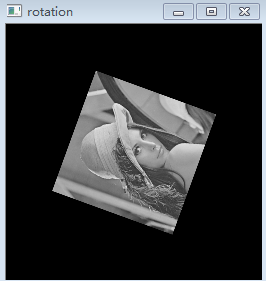展开全文• 使用matlab来实现一个图像旋转处理 一种几何变换 资源是matlab的一个m文件 代码都在里面 带有一点释放方便理解 :) 具体实现描述: http: blog csdn net abee23 article details 7398749 (支持原创哈) 测试平台...
• 以灰度图像cameraman.tif为例，利用Matlab图像处理工具箱中的imrotate函数图像进行旋转变换。要求：创建3个figure窗口，分别用于显示原始图像、逆时针旋转45°后的图像、顺时针旋转45°后的图像。并保存旋转后的...
以灰度图像cameraman.tif为例，利用Matlab图像处理工具箱中的imrotate函数对图像进行旋转变换。要求：创建3个figure窗口，分别用于显示原始图像、逆时针旋转45°后的图像、顺时针旋转45°后的图像。并保存旋转后的所有图像文件到当前目录中。
I=imread('cameraman.tif');
I_45N=imrotate(I,45,'bilinear');
imwrite(I_45N,'cameraman_nishizhen45.tif');
I_30S=imrotate(I,-30,'bilinear');
imwrite(I_30S,'cameraman_shuunshizhen30.tif');
figure(1);
imshow(I);
figure(2);
imshow(I_45N);
figure(3);
imshow(I_30S);


展开全文matlab
• 主要采用函数库的仿射变换函数图像进行旋转 关键代码如下： double scale=1;//旋转之后的缩放比例 double angle=45;//旋转角度 Point point = Point(srcImage.cols / 2, srcImage.rows / 2);//旋转中心 Mat M2 =...Opencv
• 那么，对于旋转变换呢？我们可以同样将其想象成二维平面上矢量的旋转。如下图所示，矢量[x1,y1][x_1,y_1][x1​,y1​]逆时针旋转θθθ度到了[x2,y2][x_2,y_2][x2​,y2​]。 设定矢量的长度为s，根据坐标系定义，...
• 图像的平移，旋转变换以及仿射与投影都需要先定义转换矩阵，然后使用cv2.warpAffine()根据变换矩阵完成转换 imgRotation = cv2.warpAffine(img, mat,(widthNew,heightNew),borderValue=(255,255,255)) 其中，...opencv
• 一、图像旋转变换的流程 1、确定旋转后新图像的尺寸，设原始大小为M,N 由以上公式得到： x=[0,(M-1)*cos(Angle),(M-1)*cos(Angle)+(N-1)*sin(Angle),(N-1)*sin(Angle)]; %旋转后新坐标系横坐标 y=[0,-(M-1)*sin...MATLAB
• 由几何知识和三角函数相关定理就可以求出上诉过程的数学表达式：再写成矩阵形式：其中就是旋转矩阵T与图像的缩放一样，经过上诉变化后，输出图像许多位置的灰度也无法确定，为此我们还要进行插值法处理...
• 图像旋转变换图像的位置变换，但旋转后图像的大小一般会改变。和平移变换一样，既可以把转出显示区域的图像截去，也可以扩大显示区域以显示完整的图像，如下图所示。 我们先讨论不裁剪转出部分，扩大显示区域...
• 任意角度旋转函数如下：void ImgRotate(const Mat &srcImg, Mat &rotatedImg, double degree) { int h = srcImg.rows; int w = srcImg.cols; //求对角线的长度，做一个以对角线为边长的正方形图像 int diaLength =...opencv
• 规律：（1）1.1 自变量x乘以一个大于1的数...1.3 x乘以一个小于-1的数，图像沿y轴旋转并沿x轴压缩；1.4 x乘以一个（-1,0）之间的数，图像沿y轴旋转并沿x轴伸展。 （2）2.1 因变量y乘以一个大于1的数，图像沿y轴压缩...
• 翻开任意一本图像处理的书，都会讲到图像的几何变换，这里面包括：仿射变换（affine transformation）、投影变换（projecttive transformation）。前者针对的是平面上的物体位姿变化，如水平/垂直方向位移、旋转、...opencv
• 用c++builder实现的图像的动态旋转变换。简单有效BCB
• ## Matlab图像几何变换之图像旋转

万次阅读 多人点赞 2017-12-18 16:48:18
Matlab图像旋转 ...从①②可以得到旋转变换的矩阵公式： [x1y11]\begin{bmatrix}x_1&y_1&1\end{bmatrix}=[x0y01]\begin{bmatrix}x_0&y_0&1\end{bmatrix}⎡⎣⎢cos(θ)−sin(θ)0sin(θ)cos(θ)0matlab
• 刚讲了一点"RBG转灰度图",就马上进军图像的几何变换了,同时也带来了一个新的实验(作業):. 要求:旋转弧度: 0 对于我这个"好学生"来说...当然掩盖不了心中的好奇心----图像旋转是怎么实现的?于是经过一定的探索...matlab 算法 工具
• Opencv图像旋转操作，使用cvWarpaffi,ratateImage函数opencv
• 一、图像旋转变换 1.1 图像旋转变换的理论 图像旋转变换是将一幅图像绕着某一点进行顺时针或逆时针方向旋转一定的角度，逆时针旋转为正，顺时针旋转为负。一般采用逆时针旋转。 1.2 图像旋转的步骤： （1）平...
• 对其中最后可展现完整的代码做简单记录（该代码也摘自上边链接的最后一段代码）： Mat img = imread(IMG_PATH);...//原文说要获得完整结果图像，这里变换之前的中心必须为原图的中心 degree=-7; sopencv
• 自己写的MATLAB旋转函数，方向是反向插值，方法是双线性插值
• ## 旋转变换（一）旋转矩阵

万次阅读 多人点赞 2017-03-27 17:15:37
本文主要介绍了计算机图形学中的旋转的概念和矩阵的描述方式，包括二维和三维旋转矩阵的推导过程图形
• 这里写链接内容OpenCV提供了warpAffine函数实现图片仿射变换功能，我们可以利用这个函数实现图像旋转函数原型为：CV_EXPORTS_W void warpAffine( InputArray src, OutputArray dst, InputArray M, Size dsize, ...opencv
• 1、原图像经水平、垂直、水平垂直镜像设置通过mirror函数实现： close all; %关闭当前所有图形窗口，清空工作空间变量，清除工作空间所有变量 clear all; clc; I=imread('cameraman.tif'); %输入图像 J1=......